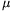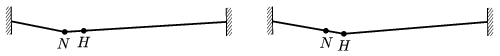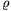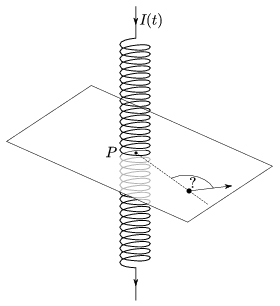Mathematical and Physical Journal
for High Schools
Issued by the MATFUND Foundation
 Already signed up? New to KöMaL?

# KöMaL Problems in Physics, November 2010

Show/hide problems of signs:## Problems with sign 'M'

Deadline expired on December 10, 2010.

M. 309. Nowadays many household devices or toys are operated with button cells. Buy two different and unused button cells and determine by measurement their

a) electromotive forces;

b) internal resistances;

c) electrical energy.

(6 pont)

statistics## Problems with sign 'P'

Deadline expired on December 10, 2010.

P. 4284. A man carries a beam on his shoulder. The centre of mass of the beam is not above his shoulder, thus he places his arm onto the beam in order to balance it. Someone states that the force which is exerted on his shoulder is greater than the weight of the beam. Is he right?

(3 pont)

solution (in Hungarian), statistics

P. 4285. The capacitance of an electrolyte condenser of a flash, which is to store energy, is 3300F, and its operating voltage is 350 V. What percent of the stored energy can be used for the flash, if the discharging process is ceased in the discharge tube at 50 V.

(3 pont)

solution (in Hungarian), statistics

P. 4286. Waves are generated from a wave source, (the phase angle is zero when they are started.) and they travel along a straight line at a speed of 1 m/s. When a point, in the wave, has zero displacement, the closest point which has the maximim displacement is at a distance of 1 m.

a) Find the wavelength and the frequency.

b) What is the distance between those points whose phase difference is 270o?

c) Measured from the initiation of the waves, when will the phase of the point 5 m from the wave-source first be 180o?

(3 pont)

solution (in Hungarian), statistics

P. 4287. An object, which decelerates uniformly along a circular path, looses two-thirds of its speed while it covers a half circle. From this stage, what fraction of the whole circular path does it cover until it stops, and how long does it take?

(4 pont)

solution (in Hungarian), statistics

P. 4288. A spring of length, is hang and when an object is attached to it, its length changes to 2. (The extension of the spring is proportional to the force and its mass is negligible.) When the object, attached to the spring, undergoes uniform circular motion along a horizontal path, the length of the spring is L What are the radius of the circle and the angular speed of the object?

(4 pont)

solution (in Hungarian), statistics

P. 4289. An ice floe is floating in the sea. The part which is above the water is a prism of height 0.5 m. A 200-kg seal climbs onto the floe, which sinks 5 cm deeper into the water. How many seals, which have the same mass, can the floe hold without sinking totally into the water? What is the volume of the ice floe?

(4 pont)

solution (in Hungarian), statistics

P. 4290. A thin, strong steel cable is stretched horizontally, with a great force, above a street. A rope dancer walks along the cable. When she covers one-fourth of the length of the cable, (she is at point N) the trisecting point of the cable (H) is 10 cm below its original position. What will the decent of the point N be, when the rope dancer is at point H? (The mass of the cable is negligible, the deflection of the rope is very small with respect to the length of the cable, and the tension in the cable can be considered constant.)(5 pont)

solution (in Hungarian), statistics

P. 4291. Two soap bubbles are stuck to each other. One half of the gained shape has a radius of R=6 cm and the other half has a radius of r=2 cm.

a) What is the sphere-radiusof the spherical cap which separates the two parts?

b) What is the difference between the pressures in the two parts? The surface tension of the soap solution is (=0.029 N/m.)

c) By what factor is R greater than r in case of two other bubbles which stick together, if the sphere-radius of the cap separating the two parts is R?

(4 pont)

solution (in Hungarian), statistics

P. 4292. The blood pressure of a healthy man is 120/80 Hgmm. When intravenous (IV) therapy is carried out, some solution is given directly to the vein. From what height should the IV solution be given in order to make the fluid flow into the artery. What is the blood pressure in the vein if the IV solution flows back when the solution bag is below the height of 40 cm?

(4 pont)

solution (in Hungarian), statistics

P. 4293. In a dry sauna the temperature is 100 oC, while in a concert hall, which has 2000-times bigger volume, the temperature is 20 oC. By what factor is the internal energy of the air in the concert hall bigger than that of in the sauna?

(4 pont)

solution (in Hungarian), statistics

P. 4294. An initially stationary, charged, pointlike object can move along a horizontal surface, without friction. Not far from the object there is a long vertical solenoid. The current in thee solenoid is uniformly increased from zero to a given value, and then it is decreased also uniformly to zero again. At the end of this process to which direction will the charged object move with respect to the point P, shown in the figure?(6 pont)

solution (in Hungarian), statistics